## Frequency Domain Representation:

Frequency Domain Representation – A very important representation of discrete time systems can be obtained in terms of sinusoidal or complex-exponential signals.

This is possible because the linear shift invariant systems have the interesting property that the response to a sinusoidal or complex exponential input is also sinusoidal or complex-exponential, having the same frequency and amplitude, and a phase which is characteristics of the system under study.

Let us consider a discrete sequence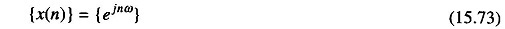defined for n = – ∞ to ∞, that is, a sampled complex exponential with radian frequency ω. The output of the system having impulse response { h(k)} can be written asLet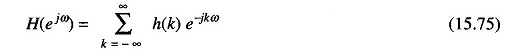we can write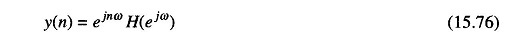The function H(e), which is called the frequency response of the system, describes the change in phase and amplitude of the input exponential, provided the series for H(e) converges. H(e) is in general a complex number, and can therefore be represented in terms of its real and imaginary parts.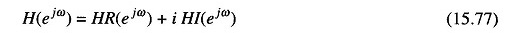or in terms of its magnitude and phase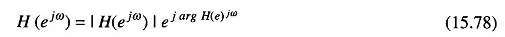In this representation the phase can be substituted by the group delay, de­fined as the negative of the first derivative of the phase with respect to ω.

### 1-D Linear Systems Described by the Difference Equation:

A very important class of linear shift invariant Frequency Domain Representation systems is the one described by the following equation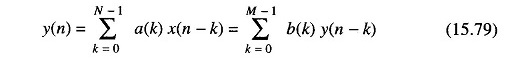where x(n) are the samples of the input sequence, y(n) are the samples of the output sequence, and a(k) and b(k) are coefficients which define the system.

In general, of course, a digital filter is not uniquely specified by the differ­ence Eq. (15.79), that is, to any solution of Eq. (15.79) we can add a component which satisfies the homogeneous difference equation (the difference equation with the LHS equal to 0) so that the overall sum satisfies Eq. (15.79).

Therefore, as with difference equations in continuous time, it is necessary to specify the initial conditions of the system. These initial conditions must be such that the system is linear and recursive.

For recursivity of the system, it is necessary that any output samples be computable from a knowledge of previously computed samples or from initial conditions.

If we take the Z-transform of both sides of Eq. (15.79), we obtain,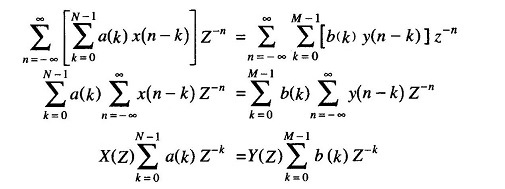from which it is possible to obtain an input-output relation in the Z-domain in the form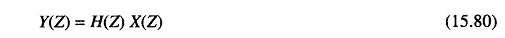where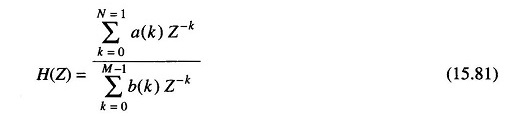Two cases can now be considered, leading to two fundamental classes of filters. If the coefficients satisfy the following condition,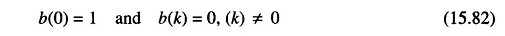the difference Eq. (15.79) reduces to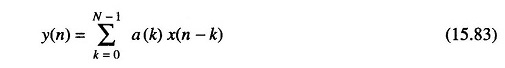and hence the transfer function reduces to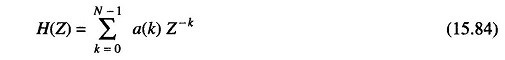In this case the output samples of the filter depend only on the input samples, without any feedback of the part output samples on the current output sample.

These filters have finite responses of length N to the unit sample sequence f(n). For this reason, they are known as Finite Impulse Response (FIR) filters, as opposed to Infinite Impulse Response (IIR) filters which are described by Eq. (15.79), where at least one (in addition to b(o) of b(k) coefficient with k ≠ 0 is different from zero.

Transfer functions of the type (15.81) and (15.84) are uniquely determined with a constant factor by the roots of the polynomials denominator and numera­tor, together with the causality condition in the IIR case.

The roots of numerator polynomials are generally called zeros of the filter, while the roots of the denominator polynomials are known as the poles of the filters. Thus FIR filters have only finite zeros, while IIR filters have both zeros and poles.

### 2-D Linear Systems Described by Linear difference Equations:

The 2-D difference equation which defines a linear shift invariant filter has the form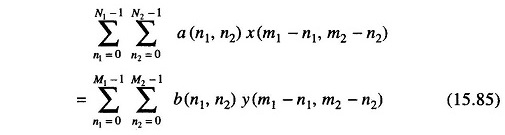where {x(n1, n2)} is the input matrix, {y(n1, n2)} is the output matrix and {a(n1, n2)}, {b(n1, n2)} are the coefficient matrices which define the transfer function of the filter.

In the (Z1, Z2) plane the filter (15.85) is described by the transfer function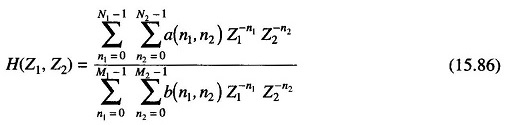If b(0, 0) = 1 and b(n1, n2) = 0 for n1 and n2 ≠ 0, Eq. (15.86) reduces to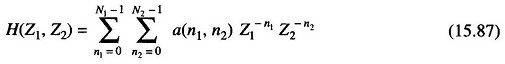With a corresponding difference equation in the form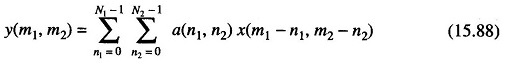As in the 1-D case, Eqs (15.87) and (15.88) define an FIR system, while Eqs (15.85) and (15.86) represent an IIR system.

In the 2-D case, it is necessary to select the choice of suitable initial condi­tions and recursivity problems, which are of greater importance due to increase in dimensionality. In the 2-D case, the problem is more involved, because the possibility of different recursive relations with zero initial conditions is increased. 2-D linear filters are further divided into quadrant recursive filters and half plane recursive filters.

Scroll to Top